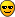# Amicable numbers

#### by Rekha 2009-12-28 14:42:59

Amicable numbers are two different numbers so related that the sum of the proper divisors of one of the numbers is equal to the other. (A proper divisor of a number is a positive integer divisor other than the number itself. For example, the proper divisors of 6 are 1, 2, and 3.) A pair of amicable numbers constitutes an aliquot sequence of period 2. A related concept is that of a perfect number, which is a number which equals the sum of its own proper divisors, in other words a number which forms an aliquot sequence of period 1.

For example, the smallest pair of amicable numbers is (220, 284); for the proper divisors of 220 are 1, 2, 4, 5, 10, 11, 20, 22, 44, 55 and 110, of which the sum is 284; and the proper divisors of 284 are 1, 2, 4, 71, and 142, of which the sum is 220.

The first few amicable pairs are: (220, 284), (1184, 1210), (2620, 2924), (5020, 5564), (6232, 636